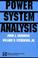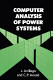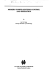### Power Systems Analysis

#### Course Description

Introduction to Power Systems Analysis. Network Equations. Network models: generators, lines and transformers, voltage control devices. Admittance and impedance matrices. Numerical methods for load flow. Gauss and Gauss-Seidel methods. Newton-Raphson and Fast Decoupled Load Flow. Sparse matrix algebra in network calculations. Simplified load flow models. DC models. 3-phase load flow. Fault analysis.

#### General Competencies

Student will gain knowledge of electric power system modeling and analysis.

#### Learning Outcomes

1. describe the procedure of determining the state vector of electrical power system
2. explain mathematical procedures of power flow and short circuit calculation
3. calculate the state vector of electrical power system
4. analyze electrical circumstances in the electric power system
5. plan the power system operation
6. estimate the power system security

#### Forms of Teaching

Lectures

Lectrures are given with the use of powerpoint presentations published on the web pages. The lectures are organized through 2 cycles. The first cycle consists of 7 weeks of lectures and 1st midter. Second cycle has 6 weeks of lectures and final exam. The lectures are given in total of 13 weeks, four hours per week.

Exams

midterm exam, final exam and oral exam

Exercises

2 hours per week. The exercises follow the lectures with practical and numerical examples. The focus is on the implementation of the solution methods.

Laboratory Work

6 laboratory exercises

Experimental Exercises

demonstration of power-flow solution programs

Consultations

Consultation term is determined on the first lecture in agreement with the students.

Structural Exercises

design and implementation of a power-flow solution and short-circuit analysis on a network model

Other

homeworks

#### Grading Method

Continuous Assessment Exam
Type Threshold Percent of Grade Threshold Percent of Grade
Homeworks 0 % 10 % 0 % 10 %
Mid Term Exam: Written 34 % 30 % 0 %
Final Exam: Written 34 % 40 %
Final Exam: Oral 20 %
Exam: Written 50 % 60 %
Exam: Oral 30 %

#### Week by Week Schedule

1. Introduction to Power Systems Analysis.
2. Network Equations.
3. Network models: generators.
4. Network models: lines and transformers.
5. Network models: voltage control devices.
6. Admittance matrix.
7. Impedance matrix.
8. Numerical methods for load flow.
9. Gauss and Gauss-Seidel methods.
10. Newton-Raphson and Fast Decoupled Load Flow.
11. Sparse matrix algebra in network calculations.
12. Simplified models.
13. DC models.
14. 3-phase load flow.
15. Fault analysis.

#### Study Programmes

##### University graduate
Electrical Power Engineering (profile)
Theoretical Course (1. semester)

#### Literature

Glenn W. Stagg, Ahmed H. El-Abiad (1968.), Computer methods in power system analysis, McGraw-HillJoseph J. Grainger, William D. Stevenson (1994.), Power System Analysis, McGraw-Hill EducationJos Arrillaga, C. P. Arnold (1990.), Computer Analysis of Power Systems, WileyAtif S. Debs (1988.), Modern Power System Control and Operation, Springer

#### General

ID 127564
Winter semester
5 ECTS
L3 English Level
L1 e-Learning
45 Lectures
30 Exercises
0 Laboratory exercises
0 Project laboratory

90 Excellent
80 Very Good
70 Good
60 Acceptable